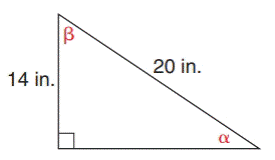Chapter 11.1, Problem 22EElementary Geometry For College St...

7th Edition
Alexander + 2 others
ISBN: 9781337614085

Solutions

Chapter
SectionElementary Geometry For College St...

7th Edition
Alexander + 2 others
ISBN: 9781337614085
Textbook Problem

In Exercises 21 to 26, find the measures of the angles named to the nearest degree.To determine

To measure:

The angle named to the nearest degree.

Explanation

In a right angle triangle,

1) The side PQ, which is opposite to the right angle PRQ is called the hypotenuse.

(The hypotenuse is the longest side of the right triangle.)

2) The side RQ is called the adjacent side of angle θ.

3) The side PR is called the opposite side of angle θ.

Formula used:

sinθ=oppositehypotenuse

Given:

By using Pythagoras theorem, that is

c2=a2+b2

Let b be the adjacent side of the triangle.

From, the diagram we have c=20 and a=14

202=142+b2400=196+b2b2=400196b2=204b=204b=14.3

For angle α, the opposite side is 14 in. and hypotenuse side is 20 in.

Then,

sin α=oppositehypotenusesin α=1420sin α=0

Still sussing out bartleby?

Check out a sample textbook solution.

See a sample solution

The Solution to Your Study Problems

Bartleby provides explanations to thousands of textbook problems written by our experts, many with advanced degrees!

Get Started

If f(4) = 10 then the point (4, _____) is on the graph of f.

Precalculus: Mathematics for Calculus (Standalone Book)

In Exercises 2528. solve the equation by factoring. 25. 8x2 + 2x 3 = 0

Applied Calculus for the Managerial, Life, and Social Sciences: A Brief Approach

Convert the following percents to decimals. 35

Contemporary Mathematics for Business & Consumers